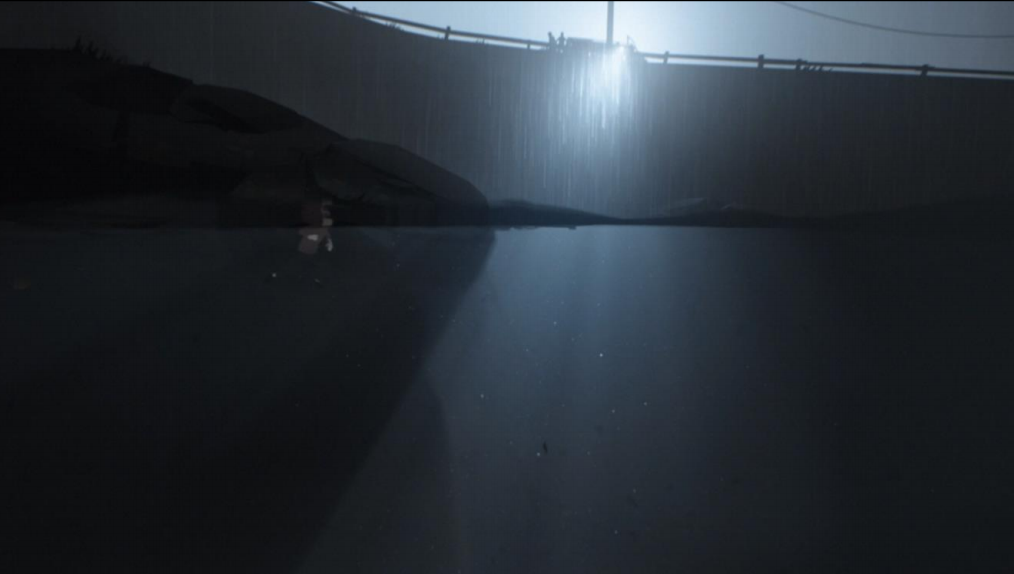## BillBoard贴片

BillBoard贴片很容易理解，用PHOTOSHOP生成一个随机的明暗条文，加上遮罩，让它看起来有光条的感觉。

## 径向模糊

1：渲染出整个画面

2：抽取出画面中高亮的部分

3：对高亮部分进行径向模糊

4：将径向模糊后的高亮层和原图合并

``````vec2 position = gl_FragCoord.xy / resolution.xy;
Position = (position-0.5)*2.;
position.y =position.y*resolution.y/resolution.x;
vec2 uv = position;
//最后颜色
vec4 color =vec4(vec3(0.),1.);
//采样次数
Const int stepNum =12;
//每次采样衰减
float decay = 0.9;
//采样权重
float weight = 1.;
//坐标移动方向
vec2 direction = normalize(uv);
for(int i=0; i < stepNum ; i++)
{
//移动坐标
uv -= direction/float(stepNum) ;
vec4 sample = texture2D(iChannel0, uv);
sample *=weight ;
color += sample;
weight *= decay;
}``````

Webgl径向模糊体积光例子

## 新的视野

PS:大厂现在做什么都号称是基于物理，实际和真正的物理学、光学比起来精度差得还是千山万水，个人觉得现在实时渲染的技术基本就和古典绘画的光影分析和制作法差不多，非要谈基于物理就有点牵强了。

``````//ro视线起点，rd是视线方向
vec3 raymarch(vec3 ro, vec3 rd)
{
const int stepNum= 100;
//光源强度
const float lightIntense = 100.;
//推进步幅
float stepSize= 250./stepNum;
vec3 light= vec3(0.0, 0.0, 0.0);
//光源位置
vec3 lightPos = vec3(2.,2.,.5);
float t = 1.0;
vec3 p = vec3(0.0, 0.0, 0.0);
for(int i=0; i<stepNum;i++)
{
vec3 p = ro + t*rd;
//采样点光照亮度
float vLight = lightIntense /dot(p-lightPos,p-lightPos);
light + =vLight;
//继续推进
t+=stepSize;
}
return light;
}``````

x(t) = ro +t*rd;

s 为光源

L(t) = I/dot(p+t*rd-s,p+t*rd-s)

L(t) = I/t^2*dot(rd,rd)+2*dot(p-s,d)t+dot(p-s,p-s)

``````float InScatter(vec3 start, vec3 rd, vec3 lightPos, float d)
{
vec3 q = start - lightPos;
float b = dot(rd, q);
float c = dot(q, q);
float iv = 1.0f / sqrt(c - b*b);
float l = iv * (atan( (d + b) * iv) - atan( b*iv ));

return l;
}``````

``````    float cosTheta = dot(lightDr,-rd);
float result = 1/(4*3.14)* (1 - g^2)/ pow(1 + g^2 -2*g* cosTheta, 1.5);``````

c是物质密度，d是距离。